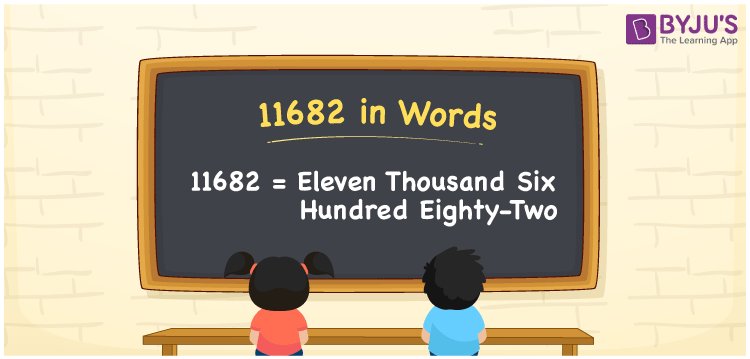# 11682 in Words

11682 in words is Eleven Thousand Six Hundred Eighty-two. The number 11682 is a five-digit number and it is a cardinal number. The place values of the number11682 are provided here using the place value system. This article will guide you to learn the procedure of writing the number 11682 in words in detail.

 11682 in Words: Eleven Thousand Six Hundred Eighty-two. Eleven Thousand Six Hundred Eighty-two in Numerical Form: 11682.

## 11682 in English Words## How to Write 11682 in Words?

Go through the below table to learn the place values of 11682.

 Ten-thousands Thousands Hundreds Tens Ones 1 1 6 8 2

The expanded form of 11682 is as follows:

= 1 × Ten thousand + 1 × Thousand + 6 × Hundred + 8 × Ten + 2 × One

= 1 × 10000 + 1 × 1000 + 6 × 100 + 8 × 10 + 2 × 1

= 10000 + 1000 + 600 + 80 + 2

= 11682

= Eleven thousand six hundred eighty-two

Hence, 11682 in words is eleven thousand six hundred eighty-two.

11682 in words – Eleven thousand six hundred eighty-two

Is 11682 an odd number? – No

Is 11682 an even number? – Yes

Is 11682 a perfect square number? – No

Is 11682 a perfect cube number? – No

Is 11682 a prime number? – No

Is 11682 a composite number? – Yes

## Frequently Asked Questions on 11682 in Words

Q1

### How to write 11682 in English words?

11682 in words is eleven thousand six hundred eighty-two.

Q2

### Simplify 11000 + 682, and express it in words.

Simplifying 11000 + 682, we get 11682. Hence, 11682 in words is eleven thousand six hundred eighty-two.

Q3

### Is 11682 a prime number?

No, 11682 is not a prime number.

Test your Knowledge on 11682 in Words